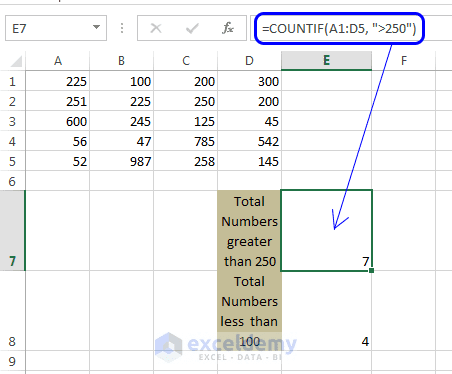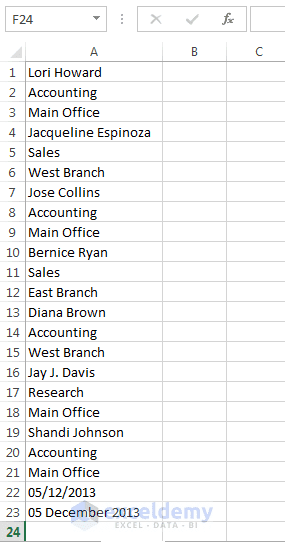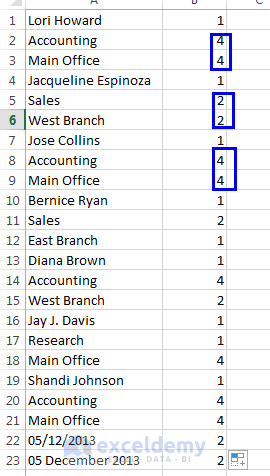Disclosure: This post may contain affiliate links, meaning when you click the links and make a purchase, we receive a commission.

# Finding out the number of duplicate rows using COUNTIF formula

Say you have a column with duplicate rows. You just want to find out which rows are duplicate and how many times they are duplicated. You can find out the duplicate ones with COUNTIF formula. We are going to show how to use COUNTIF formula and how to find out the duplicate ones using this formula. Now this system, I mean using COUNTIF formula works with the values cells contain, not the formatted values.

COUNTIF formula syntax: COUNTIF (range, criteria)

The Range is the range of cells from where you want to find your values.

The Criteria can be expressed in many ways. It can be number, expression, cell reference, or text to be fulfilled.

An Example of COUNTIF formula:` COUNTIF(A1`:D5`, ">250")`: how you will read this? Simple: Count the value if it is greater than 250 in the cell range A1 to D5.

Observe the following image. We have applied this formula: `(COUNTIF(A1: D5, ">250") )` in cell E7 and E7 displays the total numbers that are greater than 250 in the cell range A1 to D5.`COUNTIF(A1: D5, ">250")` formula we are applying in Cell E7.

## Finding out the number of duplicate rows using COUNTIF formula

We shall apply COUNTIF formula to find out the duplicate rows in a column or table. In our example, we have used a column.

First of all, let’s see the inside view of our example file. See the image below.Using COUNTIF formula to find out the duplicate rows. Inside data of our example.

1. Click B1 cell to select it. Write this formula in this cell: “=COUNTIF (A:A, A1)”. A:A means the column A and criteria A1 means: the value of cell A1. In one sentence the whole command is: Count in the value if the value is equal to A1 in column A.
2. We shall now copy this formula (“=COUNTIF (A:A, A1)”) into cell B2 to B23 using relative cell references. To do this, select cell B1. A square box will be shown in the bottom-right corner of cell B1, it is called Fill Handle. Click Fill Handle, hold it and grab until you reach cell B23.
3. Release the mouse button and you will get the following image.See the image. Some values are greater than 1. 4 is displaying after Accounting, it means Accounting has been duplicated for 4 times in column A.